数据结构list_数据结构list实现 - CSDN

• #include <iostream>...list> using namespace std; 标准类的存储方式为双向循环链表 list类 1 class list 2 { 3 list(); 4 list(int n,const T&value=T()); 5 list(T *f...
#include <iostream>
#include <list>
using namespace std;

标准类的存储方式为双向循环链表

list类

1 class list
2 {
3     list();
4     list(int n,const T&value=T());
5     list(T *first,T *last);
6     //push_back();
7     //pop_back();
8     //size();
9     //以上方法与向量相同 list中也包含
10     void push_front(const T&value);
11     void pop_front();
12
13      iterator begin();    //迭代器 默认指向第一个node
14     iterator end();        //指向表头
15
16     void erase (iterator pos);
17     void erase (iterator first,iterator last);//删区间
18     iterator insert(iterator pos,const T&value);//插在pos前
19 }

iterator(STL通用)
*iter;　　//指向节点的值
iter++;　　//指向next
iter--;
iter1==iter2;　　//指向同一个位置
*iter1==*iter2;　　//值相等 位置不一定

实例化：
std::list<int>::iterator iter=list.begin();

删除（！）：
list.erase(iter++);　　//注意++ 否则删除节点之后的表丢失 内存泄露
//此处++运算符重载后 先获取下一节点位置再删除 若++iter则先删除再获取下一位置

注意：iterator iter++=list.begin()是错误的 ++的优先级高于=而iter未赋初值

插入练习：
void doubleData(std::list <T> &aList)
{
std::list<T>::iterator p=aList.begin();
while(p!=aList.end())
{
aList.insert(p,*p);
p++;
}
}
//初始数据：1234 输出结果：11223344

删除重复元素练习：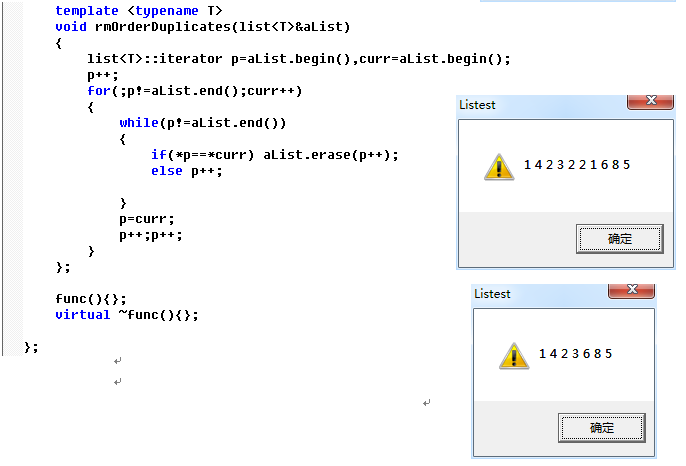转载于:https://www.cnblogs.com/verlen11/p/4187613.html
展开全文• 数据结构collection 是指数据与数据的关系，共有四种形式 （1）集合：Set，具有相同元素的总和—是关心整体的数据结构，数据与数据之间没有关系（无序的）。 （2）线性：List 数据之间具有前后关系 （3）树：Tree ...
大家好，我是被白菜拱的猪。
数据结构collection
是指数据与数据的关系，共有四种形式
（1）集合：Set，具有相同元素的总和—是关心整体的数据结构，数据与数据之间没有关系（无序的）。
（2）线性：List  数据之间具有前后关系
（3）树：Tree  数据之间具有父子关系
（4）图：Map  数据之间映射关系
今天主要介绍List
List
List线性结构：
（1）ArrayList	数组线性表			连续的内存单元存储元素 	优点：获取全部元素的速度快
与数组的区别就是数组要指定长度，ArrayList不用指定长度。
跟个自行车链条一样，可以杂乱无章的排布，每个元素存有下一个元素的地址
例外还有

Vector		向量
Stack		栈
Heap			堆
Queue		队列

其实以上介绍的用法一致，我们需要知道的是几个常用的方法
（1）尺寸size与数组的length一致，只不过在list里面换个叫法
（4）修改：set(指定位置, 新元素)
（5）获取：get（）从零开始
（6）删除：remove（）
这几个方法对于向量，栈，堆，队列都是行得通的，只不过他们是根据不同的语境使用
	List<String> l = new LinkedList<String>();

l.remove(4);

l.set(1, "new");

for (int i = 0; i < l.size(); i++) {
System.out.println(l.get(i));
}

}

List是一个借口，借口是不能创建对象的，也就是说 new List（）是错误的，因此要用实现类ArrayList实例化一个对象。
List< String >  ,<…>是泛型，告诉你这个list里面是存什么类型的值，就如数组一样，一样告诉这是一个什么样的数组，存int  还是存String。


展开全文JavaSE 新手 学习 基础 入门
• 01.第一章：List集合_List接口介绍： 1).回顾Java的集合体系结构： A).Collection(单列集合): |--List:1).有序的；2).可以存储重复元素； |--ArrayList(子类):无特有方法 |--LinkedList(子类):有一些特有方法，...
01.第一章：List集合_List接口介绍：

1).回顾Java的集合体系结构：
A).Collection(单列集合):
|--List:1).有序的；2).可以存储重复元素；
|--ArrayList(子类):无特有方法
1).push()：模拟压栈
2).poll():模拟弹栈
4).removeFirst():移除列头；
removeLast():移除列尾；
|--Set:1).无序的；2).不能存储重复元素；
|--HashSet(子类):哈希表实现：
链表 + 哈希表实现
链表：保证存储元素的顺序；
哈希表：保证存储元素的唯一性；

B).Map(双列集合):


02.第一章：List集合_List接口中的常用方法：

1).增：
public void add(int index, E ele) : 将元素ele添加到index位置。
2).删：
public E remove(int index) : 移除列表中指定位置的元素, 返回的是被移除的元素。
3).改：
public E set(int index, E ele) :用指定元素替换集合中指定位置的元素,返回值的更新前的元素。
4).查：
public E get(int index) :返回集合中指定位置的元素。
注意：上面所有方法都有一个"index(索引)"参数；这个索引在使用时要保证在正确的范围，否则抛异常；
示例代码：
public static void main(String[] args) {
List<String> list = new ArrayList<>();

System.out.println(list);

//在黄健翔的前面添加一个：孙红雷
System.out.println(list);

//移除“黄磊”
System.out.println("移除黄磊：" + list.remove(0));
System.out.println(list);

//将索引为：1的元素替换为：杨颖
System.out.println("索引为：1的元素替换为：杨颖:返回值 ： " + list.set(1,"杨颖"));
System.out.println(list);

//获取索引为2的元素
System.out.println(list.get(2));

}


03.第二章：数据结构_概念及作用：

1).什么是“数据结构”：就是指“存储和管理”大量数据的一种方式；
2).“数据结构”的作用：每种数据结构都会影响到数据的排列方式，
从而影响增、删、改、查的执行效率；


04.第二章：数据结构_数组：

1).ArrayList集合的内部就是使用“数组”结构；
2).存储方式：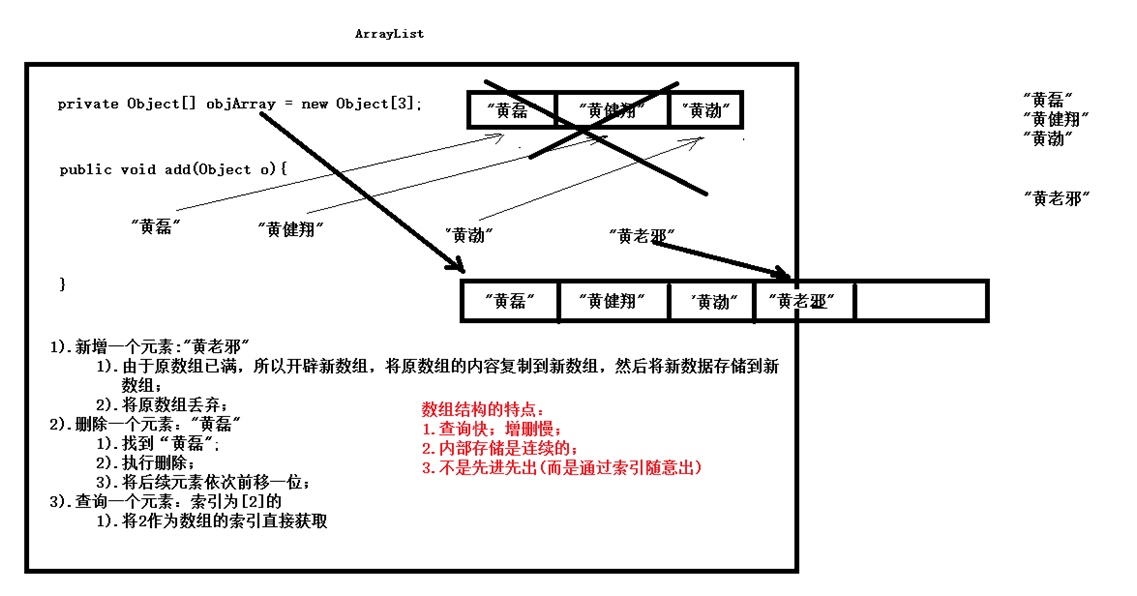3).数组结构的特点：
1).查询快；增删慢；
2).内部存储是连续的；
3).不属于“先进先出”(属于根据索引随意出)


05.第二章：数据结构_链表：

1).LinkedList内部使用的是“链表结构”：
2).存储方式：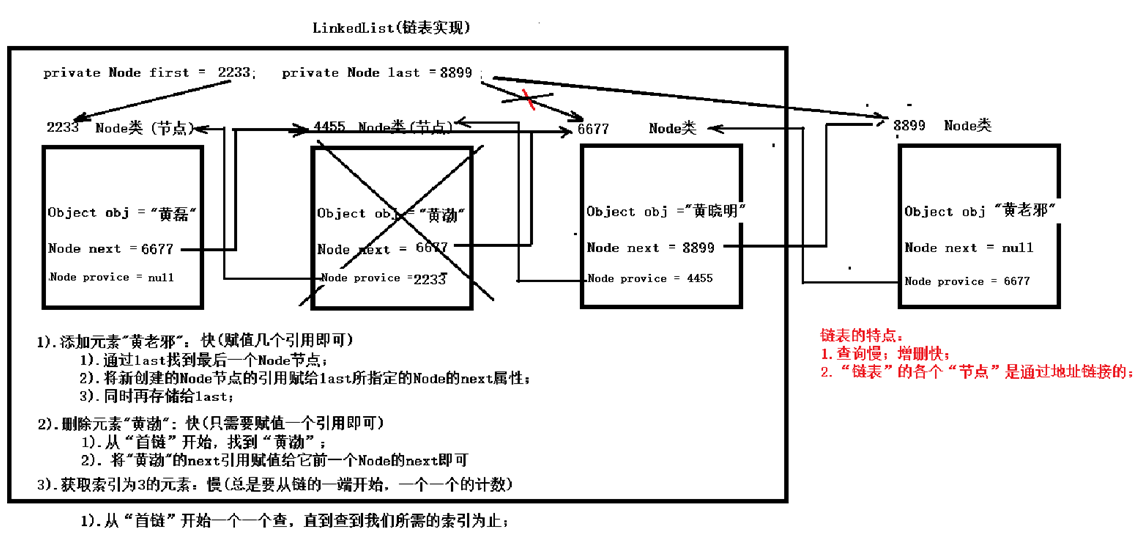3).特点：
1).查询慢；增删快；
2).各个“节点”之间通过“地址”来连接；


06.第二章：数据结构_栈和队列：

1).“栈”结构：关注“存、取”的方式：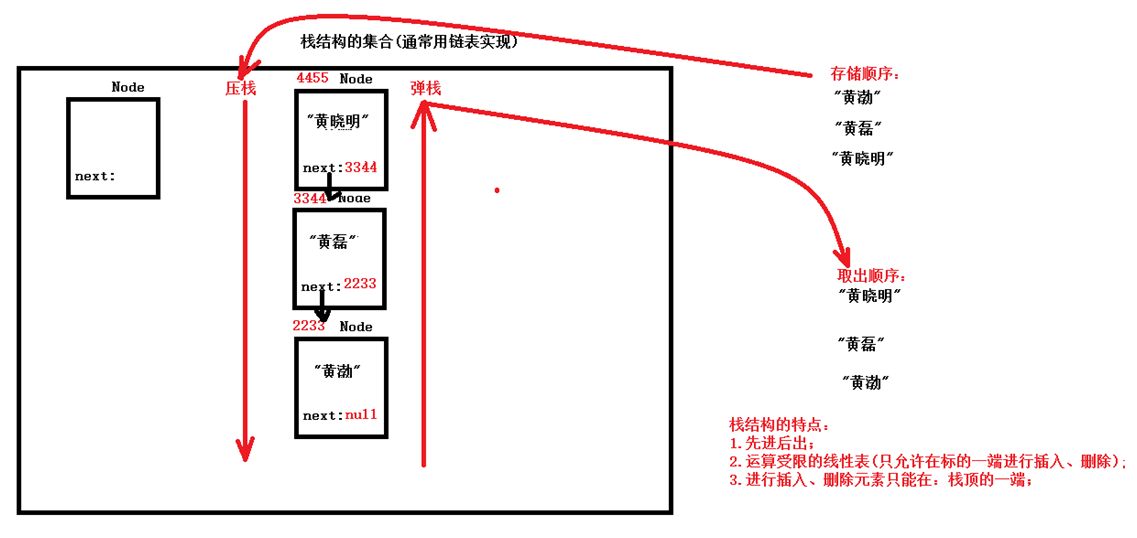特点：
1).先进后出；
2).运算受限的线性表（只能在一端进行插入、删除)
3).只能在“栈顶”进行插入和删除；
2)."队列”结构：关注“存、取”的方式：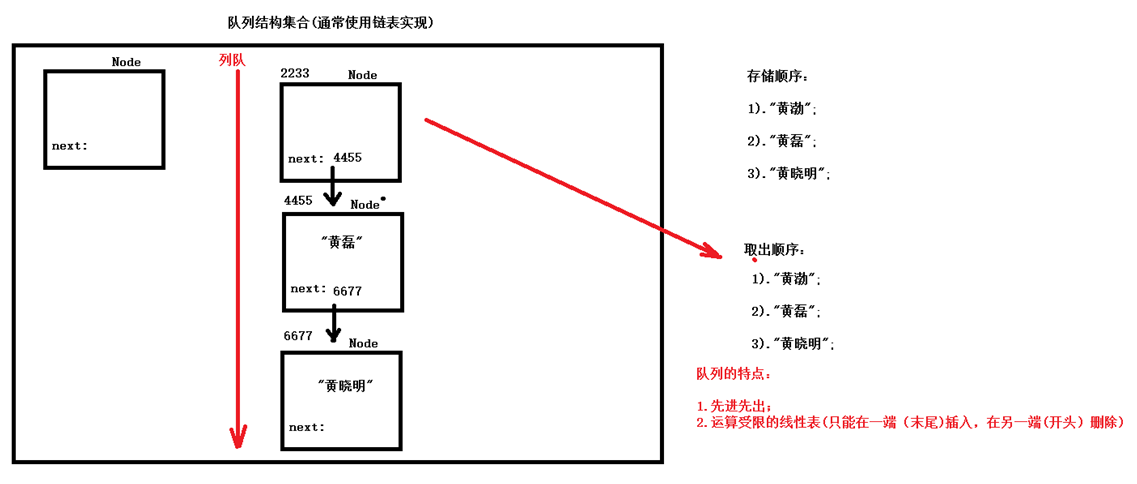特点：
1).先进先出；（必须按顺序一个一个出，不能随意出)
2).运算受限的线性表(在一端插入，在另一端删除)


07.第二章：数据结构_红黑树：

1).“树”结构：关注“存、取”的方式：
2).存储方式图示：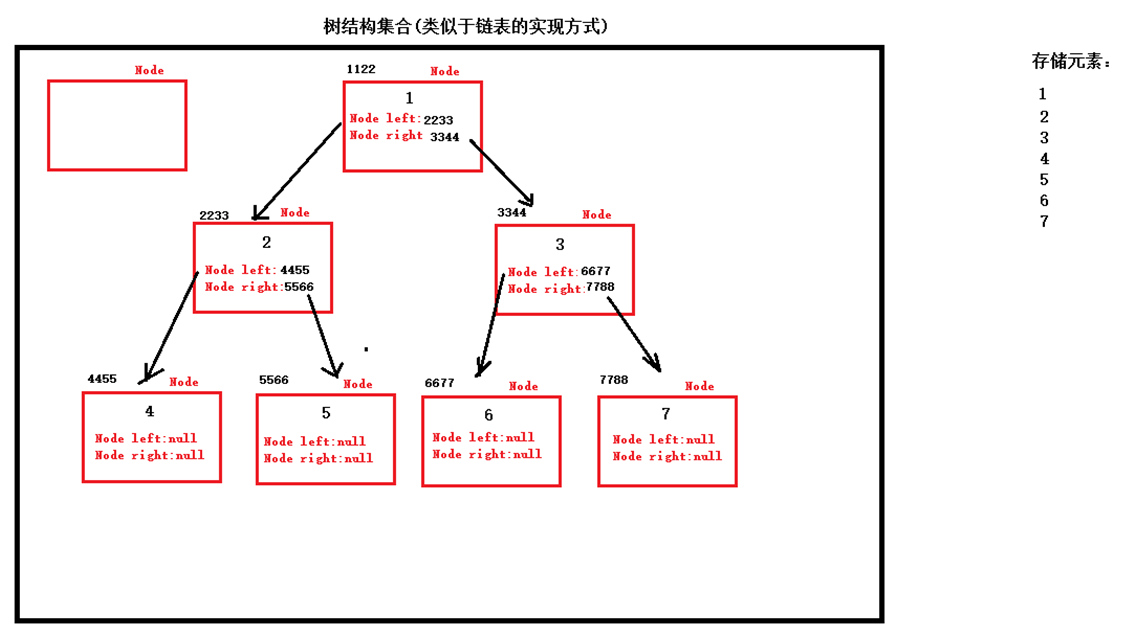08.第三章：List的子类_ArrayList集合：

1).无特有方法：
2).内部数组实现(查询快；增删慢)
3).代码：
ArrayList<String> list = new ArrayList<>();
//遍历：方式一：迭代器(foreach)Collection接口
Iterator<String> it = list.iterator();
while(it.hasNext()){
System.out.println(it.next());
}
//遍历：方式二：get(index)List接口
for(int i = 0;i < list.size(); i++){
System.out.println(list.get(i));
}


1).有一些特有方法，可以模拟栈、队列；
3).push():可以模拟压栈
4).pop():从列表的开头取出，并删除一个元素；可以模拟弹栈；
poll():从列表的开头取出，并删除一个元素；可以模拟弹栈；（如果列表为空，不抛异常，比较常用);
2).内部是“链表”实现；查询慢；增删快；
public class Demo {
public static void main(String[] args) {

//压栈
list.push("孙悟空");
list.push("猪八戒");
list.push("白骨精");
list.push("白龙马");

//弹栈
while (list.size() > 0) {
System.out.println("弹栈：" + list.poll());
}
}


}

10.第四章：Set接口_HashSet存储字符串：

public class Demo {
public static void main(String[] args) {
Set<String> set = new HashSet<>();

String s = new String("白龙马");

for(String ss : set){
System.out.println(ss);//无序
}
}


}

11.第四章：Set接口_HashSet存储自定义对象：

public class Demo {
public static void main(String[] args) {
Set<Student> stuSet = new HashSet<>();

//Student类要重写hashCode()和equals()方法

for (Student stu : stuSet) {
System.out.println(stu);
}
}


}

12.第四章：Set接口数据结构哈希表：

1).哈希表存储结构：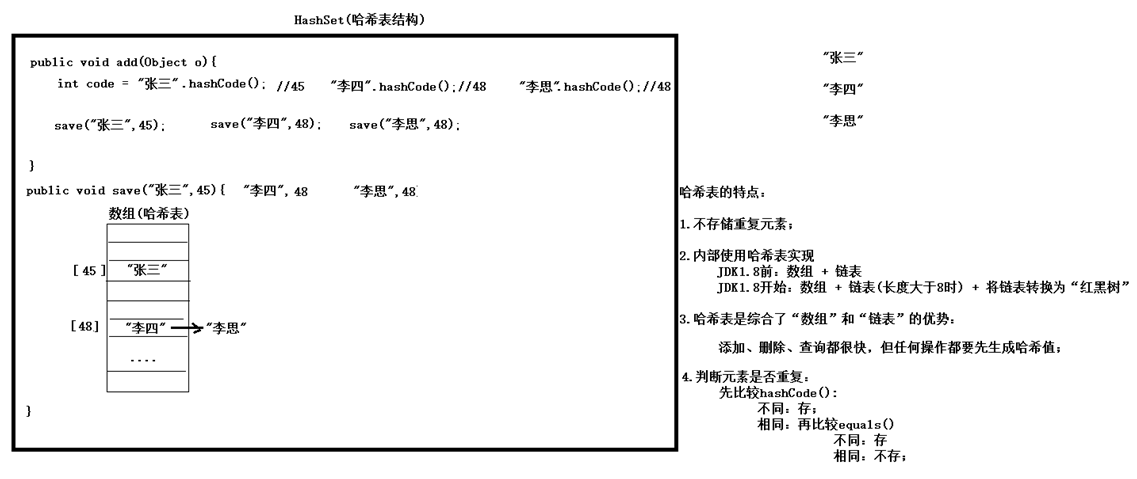1).LinkedHashSet是Set的特例：有序的
由链表 + 哈希表实现；
链表：保证元素的顺序；
哈希表：保证元素的唯一；
2).示例代码：
public class Demo {
public static void main(String[] args) {

for (String s : set) {
System.out.println(s);//有序的
}
}


}

14.第四章：Set接口_可变参数

public class Demo {
public static void main(String[] args) {

sum(10, 20);
sum(10, 20, 3, 40, 532, 43, 24, 324, 324, 324, 324, 43);
p1();//不传实参
p1("43l","fdsaf","fsjklsdjfs","fsdf");//可以传任意多的实参

}
public static int sum(int... arr) {//语法糖：编译后：就是"数组"
int sum = 0;
for(int i = 0;i < arr.length; i++){
sum += arr[i];
}

return sum;
}

public static void p1(String ... arr){

}
}
说明：
1).可变参数可以是任何类型(基本类型、引用类型);
2).调用时，对于“可变的形参”，可以不传实参，也可以传任意多的实参；
3).在一个方法的形参列表中，最多只能有一个“可变参数”。
4).在一个方法的形参列表中，可以同时出现“可变参数”和“普通参数”，但“可变参数”必须位于参数列表的末尾。
5).“可变参数”编译后就是“数组”，在方法内部，按照数组处理即可。


15.第五章：Collections工具类_常用功能：

1).java.util.Collections(工具类):里面包含了一些对Collection集合操作的工具类
2).常用方法：
1).public static <T> boolean addAll(Collection<T> c, T. . . elements) :往集合中添加一些元素。
示例代码：
ArrayList<Integer> intList = new ArrayList<>();

System.out.println(intList);

2).public static void shuffle(List<?> list) 打乱顺序 :打乱集合顺序。
示例代码：
System.out.println("打乱顺序：");
Collections.shuffle(intList);
System.out.println(intList);

3).public static <T> void sort(List<T> list) :将集合中元素按照默认规则排序（自然排序，升序）。
基于被排序对象的:compareTo()方法(需要实现Comparable接口)
示例代码：
System.out.println("排序：");
Collections.sort(intList);
System.out.println(intList);

4).public static <T> void sort(List<T> list,Comparator<? super T>):将集合中元素按照指定规则排序。
使用比较器(Comparator)可以允许被排序对象不实现Comparable接口。但是要制作一个比较器(Comparator
的子类),具体例子见：17


16.第五章：Collections工具类实现比较方式一为类添加比较功能_Comparable接口：

1).java.util.Comparable接口：作用：添加“比较的方法”--compareTo()
2).Java类库中的一些常用类：String、Integer(各种包装类)都实现了这个接口，所以可以使用Collections工具类的sort()
方法进行排序。
3).要对“自定义类”进行排序，就需要实现Comparable接口，并实现compareTo()方法。
4).示例代码：
自定义对象：
public class Student implements Comparable {
String name;
int age;
......
@Override
public int compareTo(Object o) {
Student stu = (Student)o;
return this.age - stu.age;
}
}
测试类：
public static void main(String[] args) {
List<Student> stuList = new ArrayList<>();

Collections.sort(stuList);//排序--内部调用Student的compareTo()方法。

System.out.println(stuList);


}

17.第五章：Collections工具类实现比较方式二比较器_Comparator接口：

public class Demo {
public static void main(String[] args) {
List<Student> stuList = new ArrayList<>();

Collections.sort(stuList,new Comparator(){//比较器
@Override
public int compare(Object o1, Object o2) {
Student stu1 = (Student)o1;
Student stu2 = (Student)o2;
//1.先按姓名排升序
int num = stu1.name.compareTo(stu2.name);
//2.如果姓名相同，按年龄降序排
int num2 = (num == 0 ? stu2.age - stu1.age : num);

return num2;
}
});
System.out.println(stuList);
}
}


学习目标总结：
01.能够说出List集合特点
1).有序的；
2).可以存储重复元素；
3).可以通过“索引”操作元素；
02.能够说出常见的数据结构
1).数组；
2).链表：
3).栈
4).队列；
5).树
6).哈希表；

03.能够说出数组结构特点
1).在内存中存储是“连续的”；
2).查询快；增删慢；
04.能够说出单向链表结构特点
1).查询慢；增删快；
2).通过相邻元素记录对方的地址实现；
05.能够说出栈结构特点
1).运算受限的线性表(只能在同一端操作插入、删除)
2).先进后出；
3).插入、删除都是在“栈顶”；
06.能够说出队列结构特点
1).运算受限的线性表；
2).先进先出；
3).插入、删除分别在两端；
07.能够说出Set集合的特点
1).无序的；
2).不能存储重复元素；

08.能够说出哈希表的特点
1).JDK8的哈希表实现：
数组 + 链表 + 红黑树
2).不存储重复元素
3).验证元素的唯一性：
先判断元素的hashCode():
不同：直接存；
相同：判断元素的equals()
不同：存
相同：不存；

09.使用HashSet集合存储自定义元素
Set stu = new HashSet<>();
注意：需要Student类重写hashCode()和equals()
10.能够说出可变参数的格式
public static void p1(int … 变量名){
1).可变参数编译后就是“数组”，所以可以按照数组进行操作；
2).方法形参列表中只能有一个“可变参数”，可以有普通参数，但可变参数必须位于参数列表的末尾；
}
11.能够使用集合工具类
Collections
2).shuffer():打乱顺序：
3).sort(List):对集合内的元素排序。基于元素的compareTo()
4).sort(List,Comparator):对list元素进行排序。基于比较器Comparator的。
12.能够使用Comparator比较器进行排序

public class Demo {
public static void main(String[] args) {
List<Student> stuList = new ArrayList<>();

Collections.sort(stuList,new Comparator(){//比较器
@Override
public int compare(Object o1, Object o2) {
Student stu1 = (Student)o1;
Student stu2 = (Student)o2;
//1.先按姓名排升序
int num = stu1.name.compareTo(stu2.name);
//2.如果姓名相同，按年龄降序排
int num2 = (num == 0 ? stu2.age - stu1.age : num);

return num2;
}
});
System.out.println(stuList);

}
}

展开全文Java List Set
• 本文使用c++实现list数据结构list的接口函数采用高效的实现方式，这样一方面使我加深对数据结构的理解，另一方便可以让我再复习复习c++，通过前几天写的vector数据结构的底层实现，我确实又进一步地熟悉使用C++...
本文使用c++实现list数据结构，list的接口函数采用高效的实现方式，这样一方面使我加深对数据结构的理解，另一方便可以让我再复习复习c++，通过前几天写的vector数据结构的底层实现，我确实又进一步地熟悉使用C++进行编程，，这次写list的实现过程中，我几乎没有怎么查看《c++prime》了哈哈，就是函数对象形式的遍历函数的声明格式怎么也想不起来，后来查书才明白函数的形参其实是个类的对象，只不过这个类名要在声明前指明，如下：

template<typename FuncClass> void traverse(FuncClass func);

list的实现包含两个类：listNode和list，其中listNode中主要描述了list中单个节点的内部结构，包含一个data变量和两个分别指向前节点和后节点的指针；而list类则是描述了一个list的结构及其操作，比如查看规模大小、插入新节点、删除节点、排序、查找等。

listNode接口列表
操作
功能
对象
listNode()
默认构造函数

listNode(T e, listNode<T> *p, listNode<T> *s)
构造函数，设置data，指向前后节点的指针

~listNode
析构函数

insertAsPred(const T& e)
在当前节点前插入一个新节点
节点
insertAsSucc(const T& e)
在当前节点后插入一个新节点
节点
list接口列表
操作
功能
对象
list()
默认构造函数，只初始化list的首尾哨兵节点

list(std::initializer_list<T> li)
构造函数(列表初始化方式)

list(listNode<T>* p, int n)
构造函数，拷贝指定节点及其后n个节点

list(list<T>& li)
构造函数，拷贝另一list对象

list(list<T>& li, Rank lr, Rank rr)
构造函数，拷贝另一list对象的指定区间

~list()
析构函数，手动释放哨兵及有效节点的内存空间

init()
list初始化时创建前后哨兵节点
列表
size()
返回list对象的规模
列表
display()
打印当前list中的所有元素
列表
first()
返回第一个有效节点的地址
列表
last()
返回最后一个有效节点的地址
列表
find(const T& e, int n, listNode<T>* p)
在节点p之前的n个长度范围内查找元素
列表
find(const T& e)
在整个list中查找元素
列表
search(const T& e, int n, listNode<T>* p)
在节点p之前的n个长度范围内查找元素e，返回不大于此元素的最大节点的地址
有序列表
search(const T& e)
在整个list中查找元素，返回不大于此元素的最大节点的地址
有序列表
insertAsFirst(const T& e)
插入元素作为first节点
列表
insertAsLast(const T& e)
插入元素作为last节点
列表
insertAsPred(listNode<T>* p, const T& e)
在节点P之前插入元素e
列表
insertAsSucc(listNode<T>* p, const T& e)
在节点P之后插入元素e
列表
insert(Rank r, const T& e)
在指定秩出插入元素(警告:线性复杂度)
列表
remove(listNode<T>* p)
删除指定节点
列表
clear()
清除list内所有有效节点
列表
deduplicate()
去除list内重复元素
列表
uniquify()
去除list内重复元素
有序列表
traverse(void(*func)(T &))
批量处理list内所有元素(函数指针方式)
列表
traverse(FuncClass func)
批量处理list内所有元素(函数对象方式)
列表
sort(listNode<T>* p, int n,int s)
排序接口汇总
列表
insertionSort()
插入排序法
列表
insertionSort(listNode<T>* p,int n)
对从p节点开始的n范围内的节点进行排序（插入排序法）
列表
selectionSort()
选择排序法
列表
selectionSort(listNode<T>* p, int n)
对从p节点开始的n范围内的节点进行排序（选择排序法）
列表
mergeSort(listNode<T>* p,int n)
对从p节点开始的n范围内的节点进行排序（归并排序法）
列表
重载运算符[]
下标运算符
列表
重载运算符=
赋值运算符（列表赋值方式）
列表
(1) listNode.h

#pragma once
typedef int Rank;

template<typename T> struct listNode   //节点元素模板类
{
//成员变量
T data;
listNode<T> *pred, *succ;    //定义前驱和后继指针，实现双向链表

//构造函数
listNode() {}     //构造list前后哨兵节点用
listNode(T e, listNode<T> *p = nullptr, listNode<T> *s = nullptr) :data(e), pred(p), succ(s) {}
//析构函数
~listNode(){}

//成员函数
listNode<T>* insertAsPred(const T& e);  //在当前节点前插入一个新节点
listNode<T>* insertAsSucc(const T& e);  //在当前节点后插入一个新节点
};

template<typename T> listNode<T>* listNode<T>::insertAsPred(const T& e)
{
listNode<T> *p = new listNode<T>(e, pred, this);    //更新4个指针的指向
pred->succ = p;
pred = p;
return p;
}

template<typename T> listNode<T>* listNode<T>::insertAsSucc(const T& e)
{
listNode<T> *p = new listNode<T>(e, this, succ);
succ->pred = p;
succ = p;
return p;
}

(2) list.h

#pragma once
#include "listNode.h"
typedef int Rank;

template<typename T> class list         //list结构:  [sentinel node]--[[first]...[]....[last]]--[sentinel node]
{
protected:
int _size;    //list规模

public:
//构造函数
list() { init(); }
list(std::initializer_list<T> li);  //列表初始化构造方式
list(listNode<T>* p, int n);        //拷贝构造，拷贝节点p及其后n个范围内的所有节点
list(list<T>& li) :list(li.header, li._size) {}    //拷贝构造，拷贝整个list对象
list(list<T>& li, Rank lr, Rank rr);  //拷贝构造，拷贝指定list对象的指定区间
//析构函数(只需要手动处理new的对象)
~list();

//普通成员函数
void init();    //list初始化时创建前后哨兵节点，_size置0
int size();     //返回list对象的规模
void display(); //打印当前list中的所有元素
listNode<T>* first();             //返回第一个有效节点的地址
listNode<T>* last();              //返回最后一个有效节点的地址
listNode<T>* find(const T& e, int n, listNode<T>* p); //在节点p之前的n个长度范围内查找元素e
listNode<T>* find(const T& e);                        //查找元素e
listNode<T>* search(const T& e, int n, listNode<T>* p); //在节点p之前的n个长度范围内查找元素e(要求list为有序序列，返回不大于此元素的最大节点的指针)
listNode<T>* search(const T& e);         //查找元素e(要求list为有序序列，返回不大于此元素的最大节点的指针)
listNode<T>* insertAsFirst(const T& e);  //插入元素作为first节点
listNode<T>* insertAsLast(const T& e);   //插入元素作为last节点
listNode<T>* insertAsPred(listNode<T>* p, const T& e);  //在节点P之前插入元素e
listNode<T>* insertAsSucc(listNode<T>* p, const T& e);  //在节点P之后插入元素e
listNode<T>* insert(Rank r, const T& e);                //在指定秩出插入元素(警告:线性复杂度)
T remove(listNode<T>* p);          //删除指定节点
int clear();		 //清除list内所有有效节点
int deduplicate();   //去除list内重复元素
int uniquify();      //去除list内重复元素(要求list为有序序列)
void traverse(void(*func)(T &));		    //批量处理list内所有元素(函数指针方式)
template<typename FuncClass> void traverse(FuncClass func);	//批量处理list内所有元素(函数对象方式)
void sort(listNode<T>* p, int n,int s);           //排序接口汇总
void insertionSort();        //插入排序法
void insertionSort(listNode<T>* p,int n);   //对从p节点开始的n范围内的节点进行排序
void selectionSort();        //选择排序法
void selectionSort(listNode<T>* p, int n);  //对从p节点开始的n范围内的节点进行排序
void mergeSort(listNode<T>* p,int n);       //归并排序法
//重载运算符
T& operator[](Rank r);  //下标运算符重载
list<T>& operator=(const list<T>& li); //赋值运算符重载
};

template<typename T> void list<T>::init()
{
trailer = new listNode<T>();
_size = 0;
trailer->succ = nullptr;
}

template<typename T> list<T>::list(std::initializer_list<T> li)
{
init();
for (auto iter = li.begin(); iter != li.end(); iter++)
{
p = p->insertAsSucc(*iter);
_size++;
}
}

template<typename T> list<T>::list(listNode<T>* p, int n)
{
init();
while (n--)
{
ptr=ptr->insertAsSucc(p->data);
p = p->succ;
_size++;
}

}

template<typename T> list<T>::list(list<T>& li, Rank lr, Rank rr)
{
init();
listNode<T>* p = li.first();
for (int i = 0; i < rr; i++)
{
if (i < lr)
p = p->succ;
else
{
ptr = ptr->insertAsSucc(p->data);
p = p->succ;
_size++;
}
}
}

template<typename T> list<T>::~list()
{
clear();   //清除所有有效节点
delete trailer;
}

template<typename T> void list<T>::display()
{
cout << "size:" << _size << endl;
if (_size)
{
for (Rank r = 0; r < _size; r++)
{
p = p->succ;
(r < (_size - 1)) ? cout << p->data << "," : cout << p->data;
}
cout << endl;
}
}

template<typename T> int list<T>::size()
{
return _size;
}

template<typename T> listNode<T>* list<T>::find(const T& e, int n, listNode<T>* p)    //包含p节点，n>1才能搜索
{
{
if (p->data == e)
return p;
else
p = p->pred;
}
return nullptr;
}

template<typename T> listNode<T>* list<T>::find(const T& e)
{
return find(e, _size - 1, last());
}

template<typename T> listNode<T>* list<T>::search(const T& e, int n, listNode<T>* p)   //包含p节点，n>1才能搜索
{
while ((n--) && (p != header))
{
if (p->data <= e) //返回不大于指定元素的最大节点，方便在其后面插入
return p;
else
p = p->pred;
}
return p;
}

template<typename T> listNode<T>* list<T>::search(const T& e)
{
return search(e, _size + 1, last());
}

template<typename T> listNode<T>* list<T>::first()
{
}

template<typename T> listNode<T>* list<T>::last()
{
return trailer->pred;
}

template<typename T> T& list<T>::operator[](Rank r)
{
while (r-->0)
{
p = p->succ;
}
return p->data;
}

template<typename T> listNode<T>* list<T>::insertAsFirst(const T& e)
{
_size++;
return p;
}

template<typename T> listNode<T>* list<T>::insertAsLast(const T& e)
{
_size++;
listNode<T> *p = trailer->insertAsPred(e);
return p;
}

template<typename T> listNode<T>* list<T>::insertAsPred(listNode<T>* p, const T& e)
{
_size++;
return p->insertAsPred(e);
}

template<typename T> listNode<T>* list<T>::insertAsSucc(listNode<T>* p, const T& e)
{
_size++;
return p->insertAsSucc(e);
}

template<typename T> listNode<T>* list<T>::insert(Rank r, const T& e)
{
while (r--)
{
p = p->succ;
}
return insertAsSucc(p, e);
}

template<typename T> T list<T>::remove(listNode<T>* p)
{
T e = p->data;
p->pred->succ = p->succ;
p->succ->pred = p->pred;
_size--;
delete p;
return e;
}

template<typename T> int list<T>::clear()
{
int oldSize = _size;
return oldSize;
}

template<typename T> int list<T>::deduplicate()
{
if (!_size) return 0;
int n = 0;
listNode<T>* lp;  //缓存p的前一个元素
for (int i = 0; i < _size;)
{
lp = p->pred;
if (find(p->data, _size, p->pred))   //在当前元素之前寻找，越界则退出
{
remove(p);n++;
p = lp->succ;
}
else
{
i++;
p = p->succ;
}
}
return n;
}

template<typename T> int list<T>::uniquify()
{
if (!_size) return 0;
int oldSize = _size;
while (p->succ!=trailer)   //队尾越界停止
{
if (p->data == p->succ->data)
remove(p->succ);
else
p = p->succ;
}
return oldSize - _size;
}

template<typename T> void list<T>::traverse(void(*func)(T &))
{
for (Rank r = 0; r < _size; r++)
func((*this)[r]);
}

template<typename T> template<typename FuncClass> void list<T>::traverse(FuncClass func)
{
for (Rank r = 0; r < _size; r++)
func((*this)[r]);
}

template<typename T> list<T>& list<T>::operator=(const list<T> &li)
{
clear();  //清空有效节点
if (!li._size) return *this;

while ((p = p->succ) != li.trailer)
{
lp->insertAsSucc(p->data);
lp = lp->succ;
_size++;
}
return *this;
}

template<typename T> void list<T>::sort(listNode<T>* p, int n, int s)
{
switch (s)
{
case 0:
insertionSort(p, n); break;
case 1:
selectionSort(p, n); break;
case 2:
mergeSort(p, n); break;
default:
break;
}
}

template<typename T> void list<T>::insertionSort()
{
if (_size < 2) return;
while (p != trailer)   //列尾溢出则终止
{
search(p->data, _size+1, p->pred)->insertAsSucc(p->data);
_size++;
p = p->succ;
remove(p->pred);
}
}

template<typename T> void list<T>::insertionSort(listNode<T>* p, int n)
{
if (n < 2) return;
int s = 0;
while ((n--) && (p != trailer))   //变量n次或列尾溢出则终止
{
search(p->data, s, p->pred)->insertAsSucc(p->data);
_size++;
p = p->succ;
remove(p->pred);
s++;
}
}

template<typename T> void list<T>::selectionSort()
{
if (_size < 2) return;
listNode<T> *p = first();
listNode<T> *ptr;   //缓存待删除的节点指针
for (int i = 0; i < _size; i++)   //_size次迭代
{
T min = first()->data;
p = first();
ptr = p;
for (int j = 0; j < _size - i; j++)   //内循环找最小值并插入到last位置(保证排序稳定)
{
if ((p->data) <= min)
{
min = p->data;
ptr = p;
}
p = p->succ;
}
remove(ptr);
insertAsLast(min);
}
}

template<typename T> void list<T>::selectionSort(listNode<T>* p, int n)
{
if (n < 2) return;
p = p->pred;
listNode<T> *pp = p->succ;  //迭代指针
listNode<T> *ptr;			//缓存待删除的节点指针
listNode<T> *trail = p;		//排序区间的最后一个元素,即排序区间为(p->pred,trail)
for (int i = 0; i < n+1; i++)
trail = trail->succ;
for (int i = 0; i < n; i++)			  //n次迭代
{
T min = (p->succ)->data;
pp = p->succ;
ptr = p->succ;
for (int j = 0; j < n - i; j++)   //内循环找最小值并插入到trail位置(保证排序稳定)
{
if ((pp->data) <= min)
{
min = pp->data;
ptr = pp;
}
pp = pp->succ;
}
remove(ptr);
trail->insertAsPred(min);
_size++;
}
}

template<typename T> void list<T>::mergeSort(listNode<T>* p, int n)    //[p,p_n）
{
if (n < 2) return;
//开始分裂
listNode<T>* ppred = p->pred;  //缓存待排序list的前哨兵
listNode<T>* pmi = p;  //计算中间节点
for (int i = 0; i < (n >> 1); i++)       //[p0,p1,p2] n=3 ==>  [p0] [p1,p2]
{
pmi = pmi->succ;
}
mergeSort(p, n >> 1);         //这两句递归语句表示已分离的两个子序列均已经排序完成
mergeSort(pmi, n - (n >> 1));

//开始归并（两个有序短序列==》一个有序长序列）  [pred][AAAAAAAAA][BBBBBBBBB]

//更新各端点的地址(在递归时插入和删除改变了逻辑顺序节点的实际物理地址)
p = ppred->succ;
pmi = p;  //计算中间节点
for (int i = 0; i < (n >> 1); i++)
{
pmi = pmi->succ;
}
for (Rank i = (n >> 1), j = (n - (n >> 1)); i || j;)
{
if ((i > 0) && (j == 0))   //只剩下前段list
{
i--;
ppred->insertAsSucc(p->data);
ppred = ppred->succ;
_size++;
p = p->succ;
remove(p->pred);
}
if ((j > 0) && (i == 0))    //只剩下后段list
{
j--;
ppred->insertAsSucc(pmi->data);
ppred = ppred->succ;
_size++;
pmi = pmi->succ;
remove(pmi->pred);
}
if ((i > 0) && (j > 0))     //两段list都有值，则选择最小的插在前面
{
if ((p->data) < (pmi->data))
{
i--;
ppred->insertAsSucc(p->data);
ppred = ppred->succ;
_size++;
p = p->succ;
remove(p->pred);
}
else
{
j--;
ppred->insertAsSucc(pmi->data);
ppred = ppred->succ;
_size++;
pmi = pmi->succ;
remove(pmi->pred);
}
}
}
}

`
展开全文C list 数据结构
• 数据结构列表List  List（列表） 是 Python 中使用最频繁的数据类型。 列表可以完成大多数集合类的数据结构实现。它支持字符，数字，字符串甚至可以包含列表（即嵌套）。 列表用 [ ] 标识，是 python 最通用...
• Reference：Python官方Document Tutorial ... This chapter describes some things you’ve learned about already in more detail, and ad
• 介绍python数据结构list的几个常用函数：如排序，增添元素，插值等等 1、选取任意一个或几个元素输出 注：下标从0开始，选取多个元素时，右边是开区间 2、以某一步长对列表进行抽取，重新赋值后形成新...Python list
• ## java数据结构之List

千次阅读 2018-09-07 10:03:56java List数据结构 二分查找 优化 List
• https://blog.csdn.net/xzp_12345/article/details/79251174
• using Microsoft.AspNetCore.Builder; using Microsoft.Extensions.DependencyInjection; using Microsoft.Extensions.Hosting; using Microsoft.OpenApi.Models; using Volo.Abp; using Volo.Abp.AspNetCore.Mvc;...数据结构 算法
• ## [数据结构]头插法与尾插法

千次阅读 多人点赞 2013-10-01 11:46:11
#include "stdio.h" ... //数据域 struct List *next; //指针域 } List; void TailCreatList(List *L) //尾插法建立链表 { List *s, *r;//s用来指向新生成的节点。r始终指向L的终端节点。 r = L;
• ## List集合的遍历方法

万次阅读 2020-10-13 14:02:27
集合框架：就是数据结构，主要有List数据结构，Set数据结构，Map数据结构List数据结构：数据内容无序，但数据的位置是有序的，从0开始，Set 数据结构：数据内容无序，但外置也无序，但内容不能有重复。Map数据结构...java list集合遍历
• ## 为什么要学数据结构？

万次阅读 多人点赞 2019-11-19 13:54:58
一、前言 在可视化化程序设计的今天，借助于...1) 能够熟练地选择和设计各种数据结构和算法 2) 至少要能够熟练地掌握一门程序设计语言 3) 熟知所涉及的相关应用领域的知识 其中，后两个条件比较容易实现，而第一个...数据结构 算法 线性结构 树结构
• ## Lua 常用数据结构

千次阅读 2014-08-13 17:51:27
Lua中的table不是一种简单的数据结构，它可以作为其它数据结构的基础。如数组、记录、线性表、队列和集合等，在Lua中都可以通过table来表示。 一、数组 在lua中通过整数下标访问表中的元素即可简单的实现数组。...lua
• 1:集合 2 Collection(单列集合) 3 List(有序,可重复) 4 ArrayList 5 底层数据结构是数组,查询快,增删慢 6 线程不安全,效率高 7 Vector 8 底层数据结构是数
• ## 数据结构（C语言版本）

万次阅读 多人点赞 2018-04-22 19:42:32
数据结构（C语言版本） 第1章 绪论 1.常用的数据结构类型：集合、线性、树形、图状。 2.数据结构： - 逻辑结构：数据元素之间的关系 - 存储结构：数据结构在计算机中的表示。存储结构分为：顺序存储结构和...
• 后台返回数据是一行一行的，但是前端展示要树形结构数据，所以需要我们自己处理函数了，小编在此献丑了，小写一个简便函数供大家参考，希望反馈一下。 返回数据格式 : var list= [ { name: '根目录1', id: 1, ...递归 简单
• ## java 中几种常用数据结构

万次阅读 多人点赞 2016-07-25 19:26:46
JAVA中常用的数据结构（java.util. 中） java中有几种常用的数据结构，主要分为Collection和map两个主要接口（接口只提供方法，并不提供实现），而程序中最终使用的数据结构是继承自这些接口的数据结构类。其主要的...数据结构 java
...